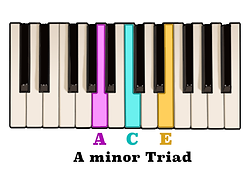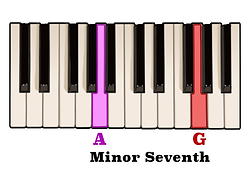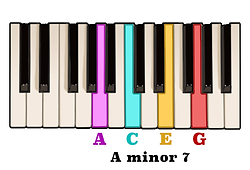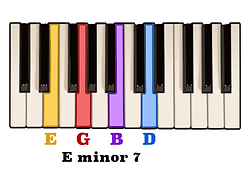top of page

## Seventh Chords | Minor Seventh Chords (2 of 5)

In the previous video we learned how the notes of a Major seventh chord consist of the notes of a Major triad with the addition of an interval of a Major seventh above the root note.

In that same respect, a minor seventh chord consists of the notes of a minor triad with an addition of a minor seventh above the root note.For example, the notes of an A minor triad are A, C, and E,and an interval of a minor seventh above the note A is the note G.Therefore, by adding the note G to the notes of an A minor triad we will have created an A minor seventh chord.In that same way, the notes of a C minor triad are C, E-flat, and G.By adding an interval of a minor seventh above the note C to the notes of a C minor triadwe would then be playing a C minor seventh chord.

Another way to think about the notes of a minor seventh chord is that they are simply derived from the first, third, fifth, and seventh scale degrees of a corresponding minor scale.

With this in mind, here are the notes of an E minor scale.We can see that the first scale degree is the note E, the third scale degree is the note G, the fifth note in the scale is the note B, and the seventh scale degree is the note D.Playing these four notes together, either melodically or harmonically, would result in an E minor seventh chord.Use this method of creating a minor seventh chord from the scale above any given root note.

Now, minor seventh chords may also be constructed by the intervals which separate each consecutive note. The pattern of intervals which produces the notes of a minor seventh chord above any given root note is minor third, Major third, minor third.Let's use this pattern of intervals to construct a minor seventh chord above the root note D.

An interval of a minor third above the note D is the note F, an interval of a Major third above the note F is the note A, and an interval of a minor third above the note A is the note C.Here we can see that the notes of a D minor seventh chord are D, F, A, and C.Use this pattern of intervals to construct a minor seventh chord above any given note.

bottom of page